Homework Help Question & Answers

# prices fluctuate in the economy. Two of the most commonly used price indexes are the consumer...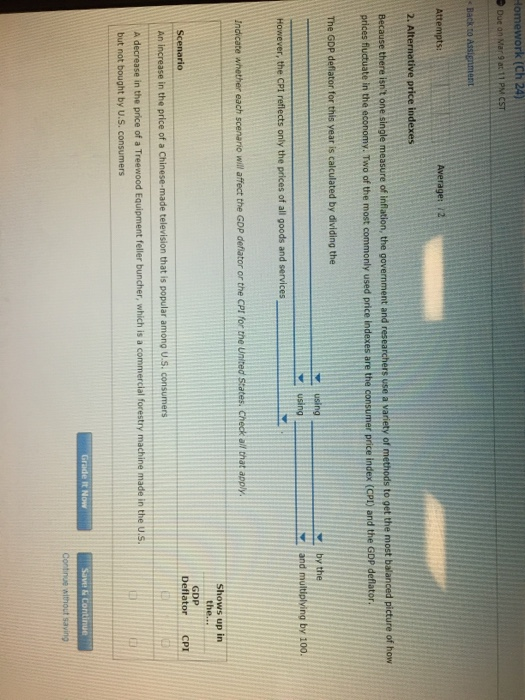prices fluctuate in the economy. Two of the most commonly used price indexes are the consumer price index (CPD and the GDP deflator using However, the CPI reflects only the prices of all goods and services Indicate whether each scenario will affect the GDP deflator or the CPI for the United states, Check all that apply Shows up in GDP Deflator CPI althout saying

#### Homework Answers

Answer #1 ✔ Recommended Answer

Ans) the answers to the blanks are 1) value of all goods and services produced in the economy this year

2) this years prices

3) value of all goods and services produced in the economy this year-

4)-the base years prices

5) bought by consumers

An increase in the price of a Chinese........... CPI

A decrease in the price of a treewood.......... GDP deflator

Add a comment
Know the answer?
Your Answer:

#### Post as a guest

Your Name:

What's your source?

#### Earn Coin

Coins can be redeemed for fabulous gifts.

Similar Homework Help Questions
• ### 2. Alternative price indexes Because there isn't one single measure of inflation, the government ...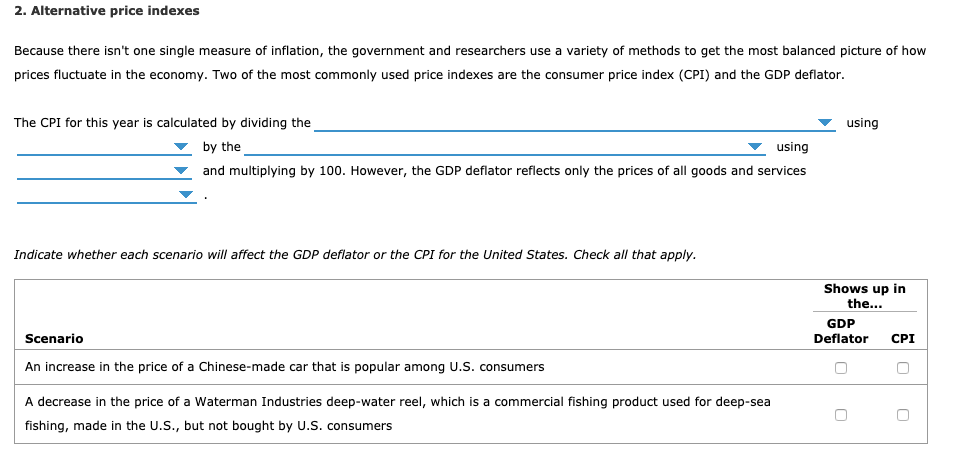1. 2. Alternative price indexes Because there isn't one single measure of inflation, the government and researchers use a variety of methods to get the most balanced picture of how prices fluctuate in the economy. Two of the most commonly used price indexes are the consumer price index (CPI) and the GDP deflator. The CPI for this year is calculated by dividing the using by the using and multiplying by 100. However, the GDP deflator reflects only the prices of all...

• ### Homework (Ch 11) Back to Assignment Attempts: 08 Average: 0.8/2 2. Alternative price indexes Because there...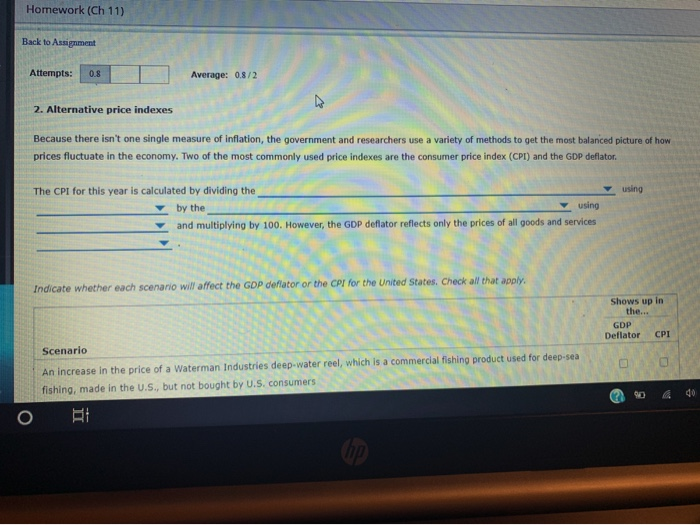Homework (Ch 11) Back to Assignment Attempts: 08 Average: 0.8/2 2. Alternative price indexes Because there isn't one single measure of inflation, the government and researchers use a variety of methods to get the most balanced picture of how prices fluctuate in the economy. Two of the most commonly used price indexes are the consumer price index (CPI) and the GDP deflator. using The CPI for this year is calculated by dividing the by the using and multiplying by 100....

• ### 2. The GDP deflator versus the consumer price index Because there isn't one single measure of...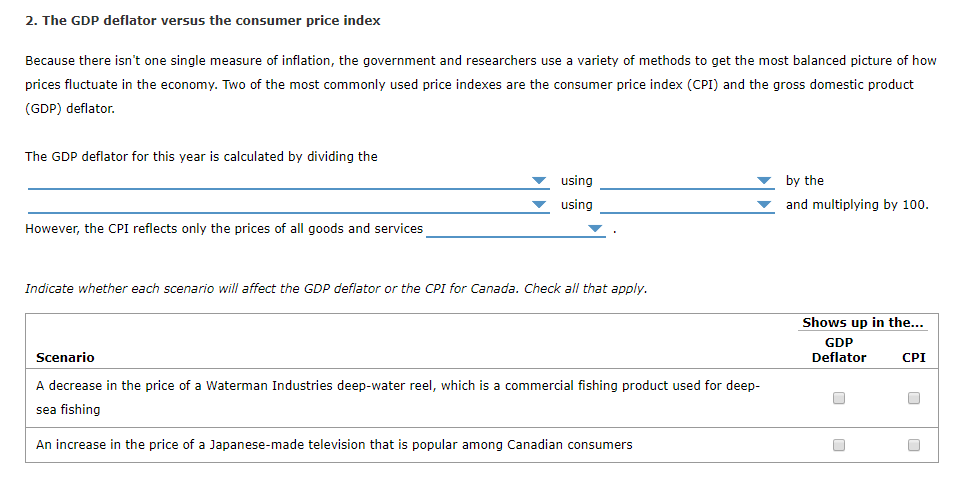2. The GDP deflator versus the consumer price index Because there isn't one single measure of inflation, the government and researchers use a variety of methods to get the most balanced picture of how prices fluctuate in the economy. Two of the most commonly used price indexes are the consumer price index (CPI) and the gross domestic product (GDP) deflator. The GDP deflator for this year is calculated by dividing the by the and multiplying by 100 USing using However,...

• ### 2. Alternative price indexes Because there isn't one single measure of inflation, the government and researchers...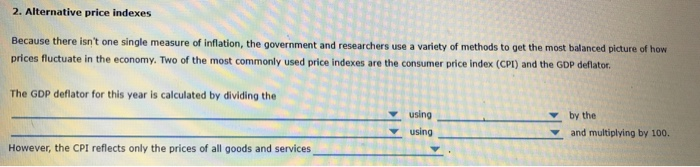2. Alternative price indexes Because there isn't one single measure of inflation, the government and researchers use a variety of methods to get the most balanced picture of how prices fluctuate in the economy. Two of the most commonly used price indexes are the consumer price index (CPI) and the GDP deflator. The GDP deflator for this year is calculated by dividing the using using and multiplying by 100. However, the CPI reflects only the prices of all goods and...

• ### 1. Calculating inflation using a simple price index Consider a fictional price index, the College...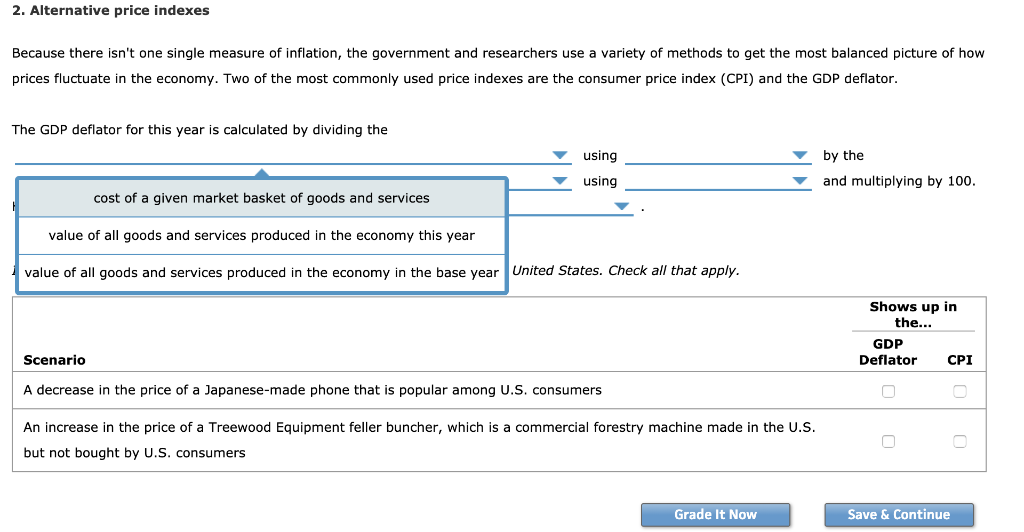1. Calculating inflation using a simple price index Consider a fictional price index, the College Student Price Index (CSPI), based on a typical college student’s annual purchases. Suppose the following table shows information on the market basket for the CSPI and the prices of each of the goods in 2014, 2015, and 2016. The cost of each item in the basket and the total cost of the basket are shown for 2014. Perform these same calculations for 2015 and 2016,...

• ### Suppose the price of thes e respond by bumer e The consumer price reflects this price...Suppose the price of thes e respond by bumer e The consumer price reflects this price increase accurately understates the price increase due to the so-called income bus overstates the price increase due to the so-called incomebas overstates the price increase due to the so-called substitution bias QUESTION 15 Laura bought word processing software in 2005 for 550. Laura's twin brother, Laurence, buys an upgrade of the same software in 2006 for \$50. What problem in the construction of the...

• ### 22. Use your knowledge of price indexes to answer the following three-part question 22a. An index...

22. Use your knowledge of price indexes to answer the following three-part question 22a. An index of prices of all domestically produced goods in the economy is the          a. Consumer Price Index. b. GDP Chain Price Index.          c. Producer Price Index. d. Wholesale Price Index. 22b. The most comprehensive (inclusive of goods) measure of the rate at which prices are changing is:          a. the CPI          b. the balance-of-payments index.          c. the GDP Chain Price Index....

• ### What is a converging economy? Match each description with the proper term. Consumer price index (CPI)...What is a converging economy? Match each description with the proper term. Consumer price index (CPI) Producer price index (PPI) Personal consumption expenditure (PCE) Gross domestic product deflator (GDP Deflator)

• ### Question 10 (5 points) Which price index comprises the prices of all final goods and services...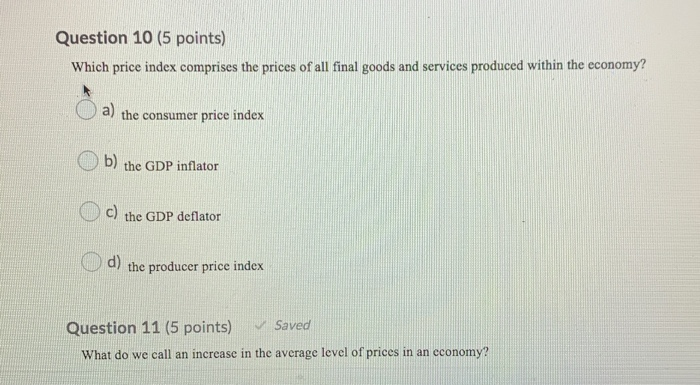Question 10 (5 points) Which price index comprises the prices of all final goods and services produced within the economy? a) the consumer price index Ob) the GDP inflator O C) the GDP deflator d) the producer price index Question 11 (5 points) ✓ Saved What do we call an increase in the average level of prices in an economy?

• ### How does the Consumer Price Index differ from the GDP deflator? Explain what is meant by...

How does the Consumer Price Index differ from the GDP deflator? Explain what is meant by the "substitution bias" in the CPI. If food prices increase by 10%, and people always spend 25% of their total consumption expenditure on food, how much will the CPI increase (all other prices stay the same)?